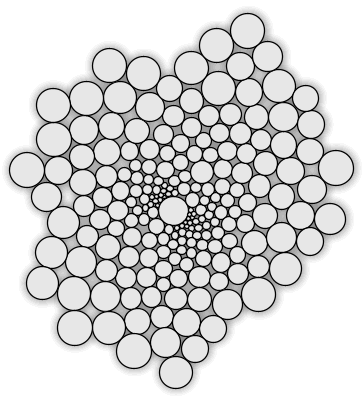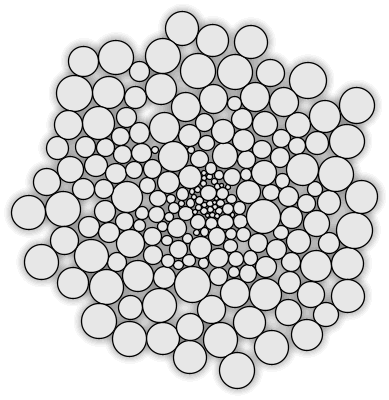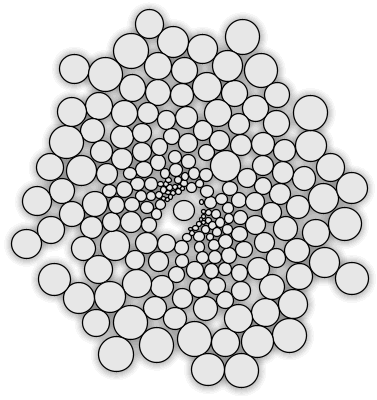# Sample: Circle Packing Plug-in (VB.NET)

Summary: A basic 2D circle packing plug-in for Rhino 4

This plug-in was made during the London 2007 DotNET workshop. It is a very simple circle packing algorithm which iteratively attempts to find a solution.

The command exposes several options, but there are no advanced features. The essential source code (VB.NET) is also available from this wiki page.

This example plug-in showcases some Rhino SDK basics such as openNURBS class inheritance and using Getters. It also provides a simple example of using display conduits to draw feedback geometry.

• The PackCircle class contains the mathematics for circle bumping.
• The PackCircles class manages a list of multiple circles.
• The PackConduit class takes care of the feedback display.

How the algorithm works: Every circle in the array is compared with all circles that have a higher index. When two circles overlap (when the distance between A and B is less than the sum of both radii) then the A circle is moved away from B (V is the translation vector), until they are touching.

Before the collision test is performed, the entire array containing all circles is sorted from the center outwards. After every collision pass, the circles move towards the basepoint to reduce the amount of wasted space. The magnitude of this motion decreases exponentially as the algorithm progresses. This plug-in comes with four different packing algorithms. The details of each differ slightly from the default behavior (Fast packing) described above.Packing as described above. Note that small circles tend to end up near the center of the pack. This solution took 140 iterations. Packing efficiency is 74%. Double packing algorithm. Here, in a collision event both circles are moved. Note the different distribution of circle sizes compared to Fast packing. This solution took 470 iterations, which is significantly slower than Fast packing. Packing efficiency = 78%.Random packing uses randomized rather than sorted collision detection. This solution took 430 iterations. Packing efficiency = 74%. Simple packing omits the contraction pass, resulting in large gaps. This algorithm is the fastest one available, but it performs very badly. This solution took 25 iterations. Packing efficiency = 68%.

Circle packing efficiency expressed as the ratio of total circle area divided by the convex hull area. This results in an underestimate of efficiency which decreases as the number of circles increases.